## Thursday, April 13, 2017

### Flip flop

j flop is an important basic memory element for digital circuit. Flip-flop is designed by assembling different logic gates. Single logic gate does not have any information storing capacity but by combining different such gates one can make such a digital circuit which can store digital information. Flip flop is such circuit. There are different types of flip flop with different characteristics for different application. Flip-flop is the basic building blocks of most sequential circuits. Flip-flop (FF), is also known as a bistable multivibrator, because it has two stable states. It y6zaætwgdxtcan remain in d of the states indefinitely. Its state can be changed ny applying the proper triggering signal. Flip flop is one-bit memory element. There are two outputs in a flip-flop generally marked as Qh and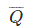. Either of Q andcan be used as output but normal practice is to take Q as output port andas inverted output port.

It is to be noted here that a flip flop has always one out complement of other output. The state of a flip-flop is normally determined by the condition of output Q. If Q = 1 the flip-flop is said to be in HIGH state or logic 1 state or SET state. When, Q = 0 the state of flip flop is said to be in LOW state or logic 0 state or RESET state or CLEAR state.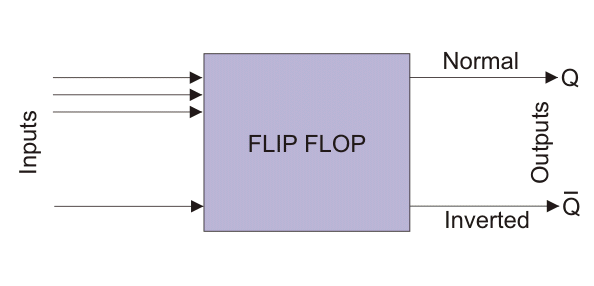The figure below shows a block diagram of a flip flop. It shows a flip-flop may have one or more inputs but only two outputs. The combination of inputs which alter the outputs or state of flip flop is referred as excitation. The excitation is used to switch the flip flop from one state to other. But the typical feature of flip flop is that once the state of flip flop is changed by applying, excitation, it remains unaltered even the excitation is removed from input ports. Hence, momentary application of excitation is enough to change the state a flip-flop. This is how flip flop behaves as memory element. When, Q = 1 it stores a 1 and when, Q = 0, it stores a 0. Flip-flops are the basic components of shift registers and counters. Flip flop is a sequential circuit hence it can be either synchronous or asynchronous. When inputs are controlled by clock pulse it is normally referred as flip flop. Here the inputs are applied but not acted until clock pulse appears and enable the inputs. When the same circuit is made asynchronous that is its inputs is not controlled by clock pulse, it is called latch. It is SET or RESET instantaneously on receiving the input signal. Latch can act independently of clock signal.

Most simple type of flip flop is S R Flip Flop. It has two inputs S and R and two outputs Q and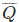. The state of this latch is determined by condition of Q. If Q is 1 the latch is said to be SET and if Q is 0 the latch is said to be RESET. This S R Latch or Flip flop can be designed either by two cross-coupled NAND gates or two-cross coupled NOR gates. When we design this latch by using NOR gates, it will be an active high S-R latch. That means it is SET when S = 1. When we design this latch by using NAND gates, it will be an active low S-R latch. That means it is SET when S = 0. S R Flip Flop is also called SET RESET Flip Flop. Figure below shows the logic circuit of S R latch.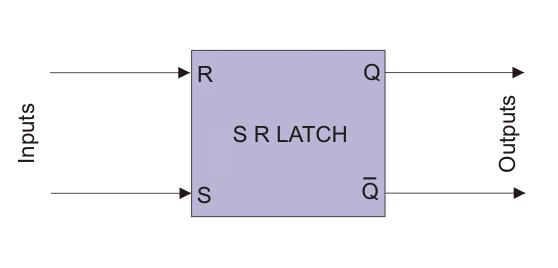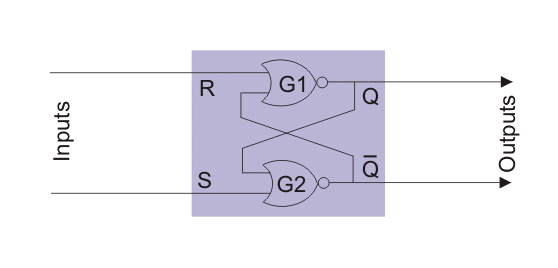In the above logic circuit if S = 1 and R = 0, Q becomes 1. Let us explain how.

NOR gate always gives output 0 when at least one of the inputs is 1.So when S is applied as 1 the output of gate G2 i.e.is 0 irrespective of the condition of second input Q to the gate.Nowis input of gate G1 so both the inputs of G1 become 0 as R is already 0. So, output of G1 is now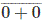or 1.So whatever may be the previous condition of Q, it always becomes Q = 1 and= 0 when, S = 1 and R = 0. This is called SET condition of the latch.In the above logic circuit if S = 0 and R = 1, Q becomes 0. Let us explain how.As we already said, a NOR gate always gives output 0 when at least one of the inputs is 1.So when R is applied as 1, the output of gate G1 i.e. Q is 0 irrespective of the condition of second inputto the gate.So, whatever may be the previous condition of Q, it always becomes 0 this 0 is then fed back to input of gate G2. As here S is already 0, both inputs of G2 are 0. Hence output of G2 i.e.will be 1. So, Q = 0 and= 1 when, S = 0 and R = 1. This is called RESET condition of the latch.In the above logic circuit if S = 0 and also R = 0, Q remains same as it was. Let us explain how.First suppose Q is previously 1.Now the inputs of G2 are 0 and 1 as S=0 and Q=1. So output of G2 i.e.is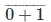or 0.Now both inputs of G1 are 0 as R=0 and=0. So output of G1 i.e. Q isor 1.Now suppose Q is previously 0.Now both inputs of G2 are 0 and 1 as S=0 and Q=0. So output of G2 i.e.isor 1.Now the inputs of G1 are 0 and 1 as R=0 and=1. So output of G1 i.e. Q isor 0.So it is proved that Q remains same as it is when S = 0 and also R = 0 in S R latch or flip flop.

In the above logic circuit if S = 1 and also R = 1, the condition of Q is totally unpredictable. Let us explain how.

First suppose Q is previously 1.Now both inputs of G2 are 1 as S=1 and Q=1. So output of G2 i.e.is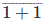or 0.Now the inputs of G1 are 1 and 0 as R=1 and=0. So output of G1 i.e. Q is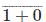or 0. That means Q is changed.Now Q is 0. So inputs of G2 are 1 and 0 as S = 1 and Q = 0. So output of G2 i.e.isor 0. That meansis unchanged.Now the inputs of G1 are 1 and 0 as R=1 and=0. So output of G1 i.e. Q isor 0. That means Q is unchanged.So, when both S and R are 1, it becomes unpredictable whether the value of output Q will be changed or unchanged. This condition of S R latch normally avoided. As the latch is SET when S = 1(HIGH), the latch is called Active High S R Latch. There is other type of latch which is SET when, S = 0 (LOW), and this latch is known as Active Low S R Latch.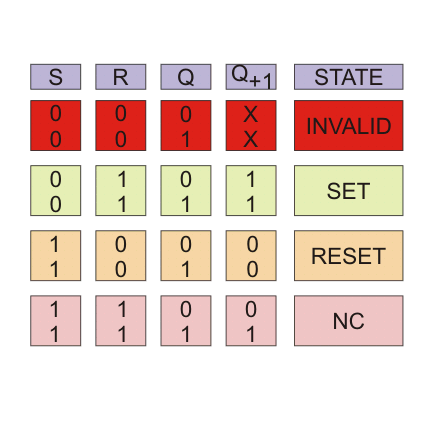### Department of Electrical Engineering (2016)

1. 05160160 APSANA SHARMA

2. 05160161 BIR MAYA RAI

3. 05160162 BIRKHA BDR. SUBBA

4. 05160163 CHEKI ZANGPO

5. 05160164 CHENCHO LHAMO

6. 05160165 CHETEN DORJI

7. 05160166 DORJI SINGYE

8. 05160167 JAMBAY CHOEDA

9. 05160168 JIGME DORJI

10. 05160169 JIMBA CHOPHEL

11. 05160170 KARMA CHODEN

12. 05160171 KARMA GAYLEG

13. 05160172 KARMA THINLEY

14. 05160173 KINLEY ZANGMO

15. 05160174 KUENLEY PENJOR
GYELTEN

17. 05160177 MON BDR GURUNG

18. 05160178 NGAWANG NAMGAY

19. 05160179 NIMA TSHERING BAL

20. 05160180 PEMA TSHERING

21. 05160181 PEMA YANGCHEN

22. 05160182 PHUNTSHO CHODEN

23. 05160183 RATU

24. 05160185 RINCHEN LHAMO

25. 05160186 SANGAY JAMTSHO

26. 05160187 SANGAY TSHERING

27. 05160188 SANJEP RAI

28. 05160189 SARDA CHUWAN

29. 05160190 SHEKHAR CHANDRA
KHATIWARA

30. 05160191 SOM BDR. RAI

31. 05160192 SONAM CHEDUP

32. 05160193 SONAM CHOKI

33. 05160194 SONAM DEKAR

34. 05160195 TASHI

35. 05160196 TASHI TENZIN

36. 05160197 TEMPA GYELTSHEN

37. 05160198 TENZIN CHENJUR

38. 05160200 TIL BDR

39. 05160201 TSHERING DORJI

40. 05160202 TSHERING WANGMO

41. 05160203 TSHEWANG DORJI

42. 05160204 TSHEWANG LHAMO

43. 05160205 UGYEN WANGCHUK

44.  05160206 VIJAY RAI

45. 05160207 YOUNTEN JAMTSHO

46. 05150173 CHOEKI DORJI

47.  05150194 NAKUL GHALLEY

## Wednesday, April 5, 2017

### Cast system

This is a system which we follow from the time when the
practice of Hindu religion started in this world. we practice it
because it is our culture that we have to follow it. Brahmin are
to give religious teaching to the people . They are to give
teaching because from the ancient time they are restricted by
religion to take intoxicated substances such as alcohol. Now
world has become so rich with people of high intelligent they
they ask reason for everything. They are so advanced with modern technologies of proving everything in practical that
they even want religious beliefs to be shown in practical field.
This system had been as far as possible by the pace of the
modernization but this system will not be eradicated
completely because if this is eradicated then Hindu religion
won't exist.

It is found that most people talks about the
practice of cast system in Hindu. And this talks
comes from only those people who don't do any
religious practice whether they be Hindu, Buddhist
or in any religion. Those who practice religious
activities (such as Rinpochoes, Pundit, lamas, and
other devotee of God) never says to eradicate this
system because they know it is not like that of
scientific theorem that is accepted only after
experimentally proven. You can't change it all of
sudden by giving drawbacks on it because it is
also one form of culture.

Now this system is changed greatly compared to
the century time ago. Nowadays people are leaving
Hinduism and Buddhism and moving towards
Christianity because it is simpler one. It Christianity
you need not to perform any ritual even when
someone dies in relative.
It doesn't matter if someone doesn't take the thing
you give thinking that one is different but if
someone doesn't give you your share thinking you
are different you have right to raise a quires.
Shekhar Khatiwara

## Sunday, April 2, 2017

### K-Map representationDigital Teaching AidKarnaugh Mapping - Lesson 4Lesson Plan(introduction...)IntroductionKarnaugh mapTruth table to Karnaugh mapPairs, Quads, and OctetsOverlapping and Rolling

PairsFig. 4-4: Four variable simplification

As you see in Fig. 4-4, only one variable goes from uncomplement to complement. Whenever this happens, you can eliminate the variable that changes form.

Proof:X = A B C

Ex:Fig. 4-5: Pairs

Whenever you see a pair first encircle it and then simplify to get the simplified Boolean expression:Quad: A group of 4 one's that are horizontally or vertically adjacent. End to end or in form of a square.

A quad eliminates two variables and their complements.

Proof:(two pairs)

X = A B (C + C)

X = A B

Encircle the quad and step through the different one's in the quad and determine which two variables go from complement to uncomplement (or vs), these are the variables that drop out.

Ex:The variables B and D can be eliminated. So we get the following equation:

X = A C

OctetFig. 4-8: Octet

An octet eliminates three variables and their complements.

Proof:X = A (C + C)

X = A

Karnaugh Simplifications

Process:

1. Draw the Karnaugh map
2. Look for octets and encircle them.
3. Look for quads and encircle them.
4. Look for pairs and encircle them.
5. Simplify and write down the equation.

Ex:Fig. 4-9: Karnaugh map### Overlapping and Rolling

Overlapping groups

Ex:Fig. 4-10: Karnaugh map

Groups can overlap to get a simpler equation:Rolling the map

Ex:Fig. 4-11: Karnaugh mapWe can roll the map and encircle a quad:HO: Simplify the following map.

Solution:HO: Simplify the following map.

Solution:Simplification Procedure for Karnaugh maps

Pair Reduction Rule : Remove the variable which changes its state from complemented to uncomplemented or vice versa.Pair removes one variable only.Quad Reduction Rule : Remove the two variables which change their states.A quad removes two variables.Octet Reduction Rule : Remove the three variables which changes their state.Octet removes three variables.Map Rolling : Map rolling means roll the map considering the map as if its left edges are touching the right edges and top edges are touching bottom edges.While marking the pairs quads and octet, map must be rolled.Overlapping Groups : Overlapping means same 1 can be encircled more than once. Overlapping always leads to simpler expressions.Redundant Group : It is a group whose all 1's are overlapped by other groups. Redundant groups must be removed. Removal of redundant group leads to much simpler expression.Ex. 1 : Represent the following boolean expression in a K-map and simplify.

F = x'yz + x'yz' + xy'z' + xy'z

Solution :

The K-map is as follows :Hence the simplified expression is

F = x'y + xy'

Ex. 2 :Simplify the following boolean expression using K-map.

F = a'bc + ab'c' + abc + abc'

Solution :

The K-map is as follows :Hence the simplified expression is

F = bc + ac'

### Earthing

Types of Earthing 1). Plate Earthing: 2). Pipe Earthing: 3). Rod Earthing 4). Earthing through the Waterman 5). Strip or Wire Earthing ...